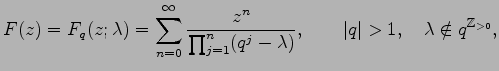# On the non-quadraticity of values of the q-exponential function and related q-series

### (25 pages)

Abstract. We investigate arithmetic properties of values of the entire functionthat includes as special cases the Tschakaloff function (\lambda=0) and the q-exponential function (\lambda=1). In particular, we prove the non-quadraticity of the numbers Fq(\alpha;\lambda) for integral q, rational \lambda and \alpha not in -\lambda qZ>0, \alpha different from 0.

The following versions are available: